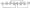# Control Systems December 2010

Note: 1. Answer any FIVE full questions, selecting at least TWO questions from each part

2. Standard notations are used„

3. Missing data, if any, may be suitably assumed.

PART-A

1    a. Define and compare open loop control systems with closed loop control systems, with examples.                                                                                                                        (06 Marks)

b. For the mechanical system shown in Fig.Ql(b), write the differential equation relating to the position y(t) and the force f(t).

C  Derive the electrical analogous quantities for the mechanical quantities using Force-voltage analogg.)

d. Derive the mathematical model for an armature controlled DC motor.       (05 Marks)

2 a. Define the transfer function. Explain Mason’s gain formula for determining the transfer function from signal flow graphs.                                                                           (06 Marks)

b.  For the block diagram shown in Fig, Q2(b), determine the transfer function using block diagram reduction algebra.Fig. Q2 (b)Fig .Q2(c)

c.   For the system described by the signal flow graph shown in Fig.Q2(c), obtain the closed loop transfer function HS. > using Mason’s gain formula.

R(s)

3 a. Define the following for an underdamped second order system :

(i) Rise time           ii) Peak overshoot              iii) Settling time.

b.  Define the steady state error coefficients. Consider a unity feedback control system whose  Determine the steady state error, when the open loop transfer function is G(s) = input is r(t) = 1 + t + at2 ; a > .0. 100 s(0.1s + l)

C. The forward path transfer function of a certain unity negative feedback control system is G(s). The system is subjected to unit step input. From the transient response curves, it is observed that the system peak overshoot is 15% and the time at which it occurs is 1.8 secs. Determine the closed loop transfer function of the system.

4 a.  Detine afesdfoalfe stability and marginal stability.

b. The Qfrem Eeao\$? transfer function of a unity negative feedback control system is given by: G(s) =       K(s + 2) s(s + l)(s + 3)(s + 5) Deteoamefe value of K at which the system is just stable.

c.   A unity feesefeek control system has: 20K where r(t) = 2t |s + l)(s + 5) + 20]? It is desnrf that for ramp input /(t)ss < 1.5. What minimum value must K have for this condition to> fee satisfied? With this K, is the system stable?

PART-B

5 a.  Define and oKglain the significance of angle and magnitude condition, as applied to the root locus method^ stability analysis of linear system.

b.  Define brafe away / in point on a root locus. Explain any one method of determining the same.

c.   For the following characteristic polynomial s2 + 2s + 2 + K(s +1) = 0, draw the root locus for 0 < K < o(08 Marks)

6  a. Explain.Nytpssfc’s stability criterion.

b.  The open loop transfer function of a unity negative feedback system is given by:b K(s + 3) G(s) = s(s -f- 2s + 2) Using the Nycpist criteria, find the value of K for which the closed loop system is just stable.

C .Derive an expression for the resonant frequency and resonant peak for a closed loop system having a second; order transfer function. For the system having open loop transfer function given by :

10(1 +0.125s) s(l + 0.5s)(l +0.25s)

7 a. Draw the asymptotic Bode magnitude and phase plots. Also determine the phase and gain crossover frequencies and gain and phase margins. Comment on closed loop stability.

b. For the Bade pfet shown, evaluate the transfer function. Refer Fig.Q7(b).Fig.Q7(b)

Fig-Q 8(a)

8 a. Obtain the state and output equation for the electrical network shown in Fig.Q8(a):

b. The state model of the system is given by :

 X] r & 11 V + “o’ u(t), Xi(0) “0“ L-3-4J _X2_ ! _x2(2)_

Where u(t) = 0 for t < 0 = ewt for t > 0 Obtain the response of the system.